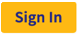Always login to Rguroo through the Rguroo’s main website at https://www.rguroo.com: To sign-in, use thebutton on the top-right of the page. This ensures that you get the latest version of Rguroo, and the Rguroo application gets loaded into your browser correctly.

In addition to routine bug fixes, the following are major additions to the Version 1.8.13:

An R console is added to the Quick Access tools, so you can execute R code. While this is an external tool, it conveniently opens in Rguroo tabs within the Rguroo working space.

### Data toolbox

#### Factor/Categorical and label/ID

Changes have been made to definitions of ID/Label and Factor/Categorical variables. The following rules are applied to classify variables when data are initially uploaded to Rguroo:

• If a dataset has 25 or fewer cases, all categorical variables are classified as Factor/Categorical.
• If a dataset has more than 25 cases and at most 100 cases, Factor/Categorical variables whose number of levels is equal to the number of cases are classified as Label/ID, and otherwise, they are classified as Factor/Categorical. -If a dataset has more than 100 cases, any Factor/Categorical variable with more than 100 levels is classified as Label/ID and any categorical variable with 100 or fewer levels is classified as Factor/Categorical
When changing attributes of a variable in the Variable Type Editor, any variable classified as Factor/Categorical can be reclassified as Label/ID. - Any variable classified as Label/ID can be reclassified as Factor/Categorical as long as its number of levels is at most 100.

#### Dataset Repository

Datasets associated with the following textbooks have been added to the Rguroo Dataset repository and are available within the Rguroo application:

• Beginning Statistics, Warren et al., Hawkes Learning
• Biostatistics: A Foundation for Analysis in the Health Sciences, 11th Edition, Wayne Daniel and Chad Cross, Wiley
• Discovering Statistics and Data, James Hawkes, Hawkes Learning
• Elementary Statistics, Navidi and Monk, McGraw Hill
• Introduction to Statistical Investigations, 2nd Edition, Tintle, et al., Wiley
• Statistics: Unlocking the Power of Data, 3rd Edition, Lock, et al., Wiley

### Analytics Toolbox

#### Simple Regression and Tests of Slope and Correlation

While simple regression can be performed in Rguroo’s general Regression function, a separate simple regression function is added to provide an easy user interface to fit simple regression models. The following are what is included in the simple regression function:

• Diagnostics plots
• Options to compute predicted values and residuals for internal and external data
• Test of hypothesis for slope and correlation using theoretical as well as permutation test.
• Confidence intervals for slope and correlation both theory-based and using bootstrap.

#### Numerical Summaries (Univariate and Multivariate)

A function called Summarization (Numerical) is added to provide univariate and multivariate summaries for numerical variables. Univariate summaries include various measures of center, spread, and shape. Moreover,you can compute any desired sample quantiles and truncated mean. The multivariate summary statistics include correlation and covariance matrix and Cronbach Alpha.

#### Other Updates to Analytics Functions

• Added the option to include prediction and confidence interval bands to the simple regression scatterplots that appear in the output.
• Added confidence bands to the QQ-plots in the regression function.
• In mean inference’s simulation plots moved the number of replications and replication seed, outside of the graph, to the text above the graph.
• In proportion inference (one population) added standard error to the confidence interval table.
• In proportion inference (two-population) added standard error to the confidence interval table.
• In proportion inference (one population) test of hypothesis added standard error and critical values to the test of hypothesis table.
• In proportion inference (two population) test of hypothesis added standard error and critical values to the test of hypothesis table.

### Plot Toolbox

#### Bubble Plot

A function is added to create bubble plots. In addition to the usual Rguroo graph customizations, this function has the following capabilities:

• Create bubble plots by factor with each level of factor being identified by a combination of colors, and plot characters circles or squares.
• Use color spectrum constructed with up to four colors to display values using color intensities.
• Jitter points in the x or y directions
• Identify outliers and identify specific cases

#### Dotplot

A rounding option was added to the Dotplot function, which allows rounding of numbers in variables to a desired number of decimal places before a dotplot is created.

#### Legends

Two options of Text Size and Point Size were added to the Legend properties. This will allow for changing the size of the point characters and the texts in the legend independently.